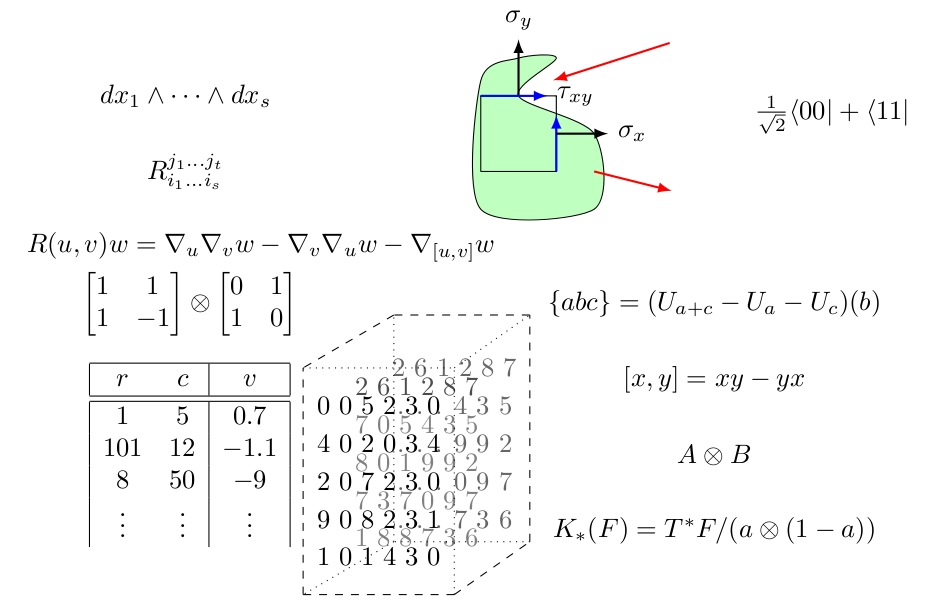# The Waring Support Rank of the Elementary Symmetric Polynomials

Definition. The Waring rank of a homogeneous polynomial $f \in C[x_1, \ldots, x_n]_d$ is the minimum $r$ such that

$f = \ell_1^d + \cdots + \ell_r^d$

for some linear forms $\ell_1, \ldots, \ell_r \in C[x_1, \ldots, x_n]_1$.

Problem. Give asymptotic bounds on A(n,d), the minimum Waring rank among all polynomials in $C[x_1, \ldots, x_n]_d$ whose support is the set of all squarefree monomials of degree $d$ in $n$ variables.

I know that $$2^{d-1} \le A(n,d) \le O(\min(6.75^d, 4.075^d \log n)).$$

If you have additional questions, feel free to reach out to a maintainer / contributor on the contact page.

#### Recovering Information in Noise

Cristopher Moore Santa Fe Institute

#### What Is a Tensor? What is a Tensor Space?